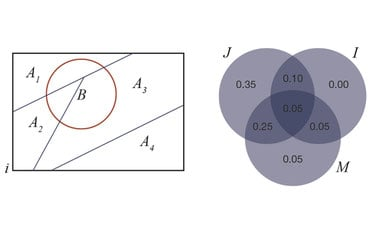Probability and Statistics I: A Gentle Introduction to Probability

This course provides an introduction to basic probability concepts. Our emphasis is on applications in science and engineering, with the goal of enhancing modeling and analysis skills for a variety of real-world problems.10,150 already enrolled! After a course session ends, it will be archivedOpens in a new tab.
Starts Jan 24
Ends May 14
Starts May 16
Ends Aug 13
Estimated 3 weeks
6–10 hours per week
Self-paced
Free

This course provides an introduction to basic probability concepts. Our emphasis is on applications in science and engineering, with the goal of enhancing modeling and analysis skills for a variety of real-world problems.

In order to make the course completely self-contained (and to bring back long-lost memories), we’ll start off with Bootcamp lessons to review concepts from set theory and calculus. We’ll then discuss the probability axioms that serve as the basis for all of our subsequent work – what makes probability tick? That discussion will give us the tools to study elementary probability counting rules, including permutations and combinations. We’ll use these rules to work on various cool applications, including poker probability calculations and baseball line-ups!

The next venues on our tour are the concepts of independence and conditional probability, which allow us to see how the probabilities of different events are related to each other, and how new information can be used to update probabilities. The course culminates in a discussion of Bayes Rule and its various interesting consequences related to probability updates.

At a glance

• Institution: GTx
• Subject: Data Analysis & Statistics
• Level: Intermediate
• Prerequisites:

You will be expected to come in knowing a bit of set theory and basic calculus. But don’t worry too much – we’ll provide bootcamps on that material in order to make the class pretty much self-contained. In addition, this course will involve a bit of computer programming, so it would be nice to have at least a little experience in something like Excel, just to bring back the programming memories.

What you'll learn

Skip What you'll learn

Upon completion of this course, learners will be able to:

• Recall Bootcamp lessons based on set theory and calculus
• Understand underlying probability axioms
• Apply elementary probability counting rules, including permutations and combinations
• Implement the concepts of independence and conditional probability
• Determine how to update probabilities via Bayes Rule

Syllabus

Skip Syllabus

“FCPS” refers to the free text, A First Course in Probability and Statistics: free access is provided via a PDF file or as a book

Module 1: Course Introduction + Bootcamps
• Lesson 1: Introduction to Probability and Statistics (FCPS §1.1)
• Lesson 2 [OPTIONAL]: The Joy of Sets Bootcamp (FCPS §1.2.1)
• Lesson 3 [OPTIONAL]: Calculus Bootcamp: Introduction + Derivatives (FCPS §1.2.2)
• Lesson 4 [OPTIONAL]: Calculus Bootcamp: Integration and Beyond (FCPS §1.2.2)
Module 2: Getting Started with Probability
• Lesson 1: Experiments, Sample Spaces, and Events (FCPS §§1.3.1−1.3.2)
• Lesson 2: What is Probability? (FCPS §1.3.3)
• Lesson 3: Basic Probability Results (FCPS §1.3.3)
• Lesson 4: Finite Sample Spaces (FCPS §1.4)
• Lesson 5: Counting Techniques: Baby Examples (FCPS §1.5.1)
• Lesson 6: Counting Techniques: Permutations (FCPS §1.5.2)
• Lesson 7: Counting Techniques: Combinations (FCPS §1.5.3)
• Lesson 8: Hypergeometric, Binomial, and Multinomial Problems (FCPS §§1.6.1−1.6.3)
• Lesson 9: Permutations vs. Combinations (FCPS §1.6.4)
• Lesson 10: The Birthday Problem (FCPS §1.6.5)
• Lesson 11: The Envelope Problem (FCPS §1.6.6)
• Lesson 12: Poker Problems (FCPS §1.6.7)
• Lesson 13: Conditional Probability (FCPS §1.7.1)
• Lesson 14: Independence Day (FCPS §1.7.2)
• Lesson 15: Partitions and the Law of Total Probability (FCPS §1.8)
• Lesson 16: Bayes Theorem (FCPS §1.8)